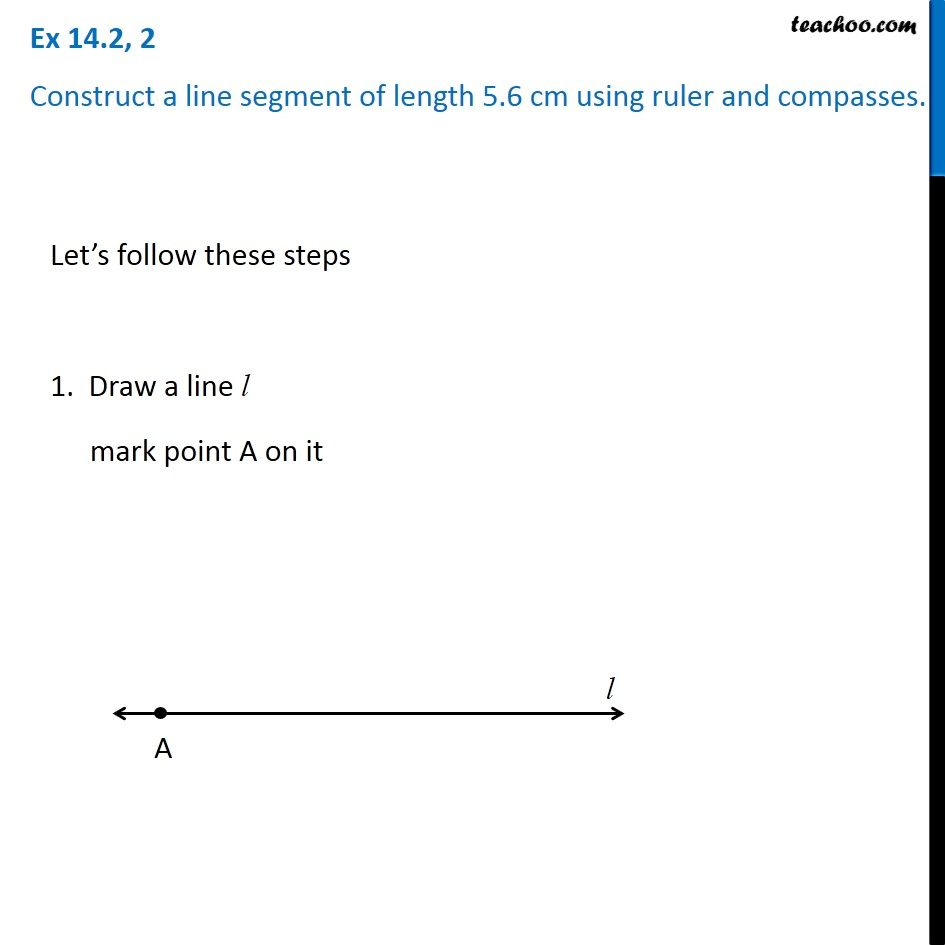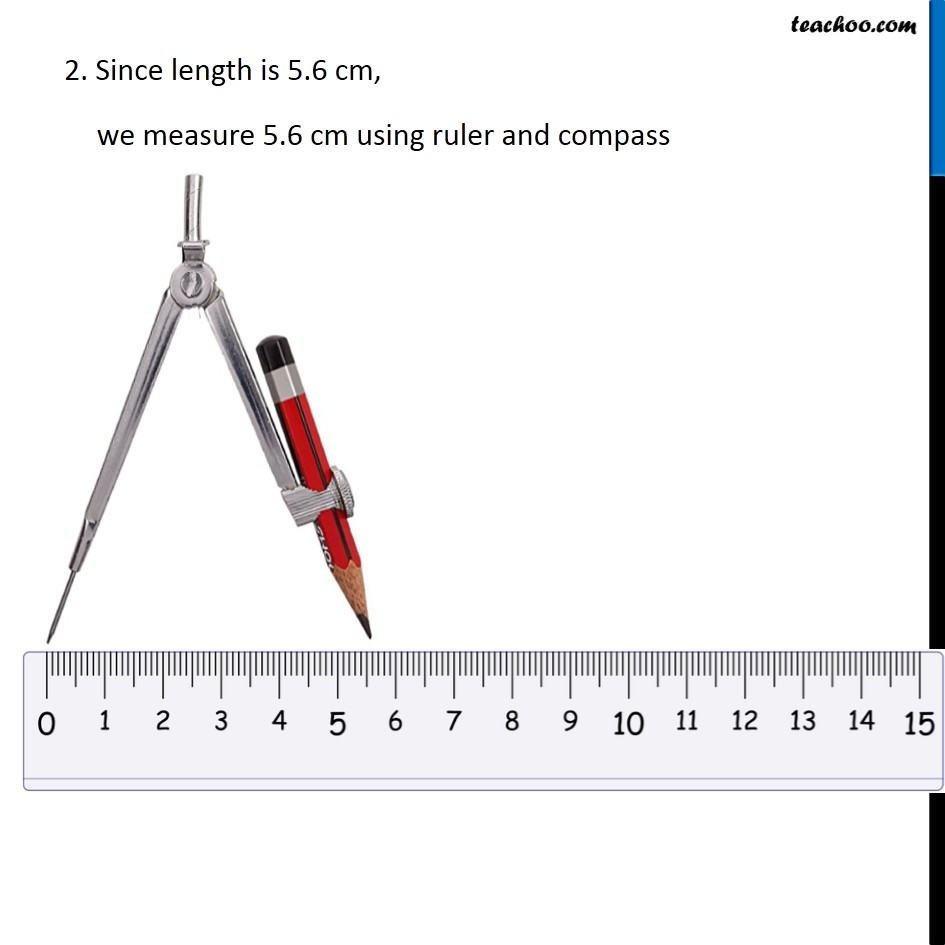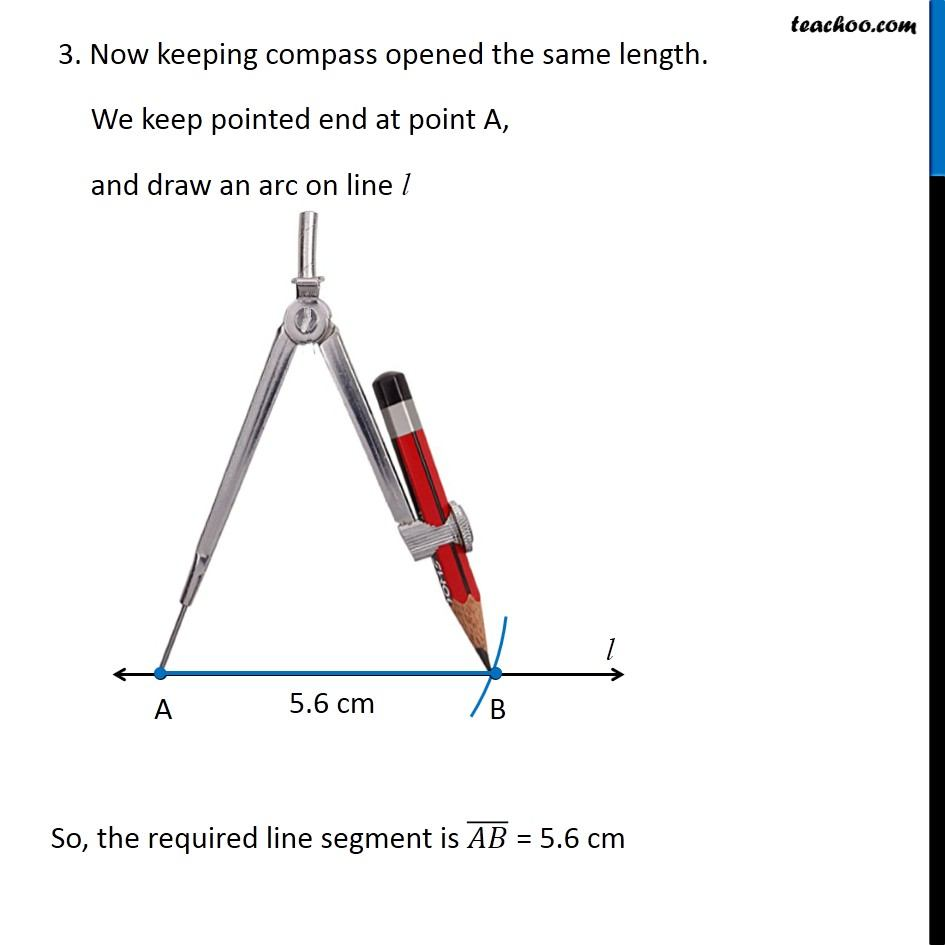Constructing a line segment

Practical Geometry
Serial order wiseLearn in your speed, with individual attention - Teachoo Maths 1-on-1 Class

### Transcript

Question 2 Construct a line segment of length 5.6 cm using ruler and compasses. Let’s follow these steps 1. Draw a line l mark point A on it 2. Since length is 5.6 cm, we measure 5.6 cm using ruler and compass 2. Since length is 5.6 cm, we measure 5.6 cm using ruler and compass 2. Since length is 5.6 cm, we measure 5.6 cm using ruler and compass So, the required line segment is (𝐴𝐵) ̅ = 5.6 cm